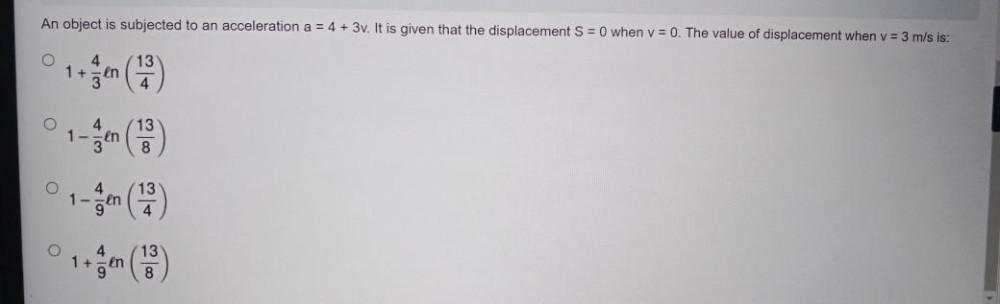Question:

# An object is subjected to an acceleration a = 4 + 3v. It is given that the displacement S = 0 when v = 0. The value of displacement when v = 3 m/s is: 1. 1+(4/3)ln(13/4) 2. 1-(4/3)ln(13/8) 3. 1-(4/9)lAn object is subjected to an acceleration a = 4 + 3v. It is given that the displacement S = 0 when v = 0. The value of displacement when v = 3 m/s is: 1. 1+(4/3)ln(13/4) 2. 1-(4/3)ln(13/8) 3. 1-(4/9)ln(13/4) 4. 1+(4/9)ln(13/8)